Checkout JEE MAINS 2022 Question Paper Analysis : Checkout JEE MAINS 2022 Question Paper Analysis :

# Orbitals

## What are Orbitals?

Orbitals in Physics and Chemistry is a mathematical function depicting the wave nature of an electron or a pair of electrons present in an atom. The probability of finding an electron around the nucleus can be calculated using this function. In simpler terms, atomic orbital can be described as the physical bounded region or space where the electrons are present. Generally, an atom consists of electrons that are fixed inside the electronic orbitals.

## Atomic Orbitals and Quantum Numbers

If we look at any atomic orbital, it is generally associated with three quantum numbers. That being said, Schrödinger’s equation solution also provides the possible energy levels that electrons can occupy and the associated wave function(s) (ψ) corresponding to each energy level. Each quantized energy states are characterized by a set of three quantum numbers n, ℓ, and ml depicting the energy, angular momentum, shape.

An atomic orbital is known as the wave function ψ for an electron in an atom. Nonetheless, the quantum numbers help us to clearly describe the arrangement of the electrons in a particular atom.

1. The principal quantum number ‘n’ is a positive integer having the values 1,2,3……The principal quantum number tells the size and energy of the orbital. All the orbitals of ‘n’ contains a single shell of an atom.
2. Azimuthal quantum number ‘l’ is referred to as subsidiary quantum number or orbital angular momentum number and defines an orbital’s three-dimensional shape.
• Each shell constitutes one or more than one subshells or sub-levels. The number of subshells in a principal shell is equal to the value of n.
• Different value for l : 0 1 2 3 4 corresponds to s p d f g orbitals respectively.

3. Magnetic orbital quantum number ‘ml’ describes an orbital’s spatial orientation in accordance with the co-ordinate axis.

Electron spin ‘s’ is a spin angular momentum of the electron. There are two orientations which describe the spin values +½ or –½. These are known as the two spin states for an electron.

Meanwhile, the size, as well as the shape of orbitals, is usually determined from the square of the wave function Ψ2.

## Types of Orbitals

Below we will look at some of the common types of orbitals and discuss a few things about orbital shapes.

### s Orbital

S orbital is spherically symmetrical orbital around the atomic nucleus. The energy level increases as we move away from the nucleus, therefore the orbitals get bigger. The order of size is 1s< 2s< 3s<.

The probability of finding an electron is maximum in 1s and decreases rapidly as we move away from it. In the case of 2s orbital, the probability density decreases sharply to zero and again starts increasing. On reaching a small maximum it decreases again and finally reaches to zero if the value of r increases further.

The nodal point is a point at which there is a zero probability of finding the electron. There are two types of nodes: Radial nodes and angular nodes. The radial nodes calculate the distance from the nucleus while the angular node determines direction.

No. of radial nodes = n – l – 1

No. of angular nodes = l

Total number of nodes = n – 1

Nodal planes are defined as the planes of zero probability region to find the electron. The number of planes is equal to l.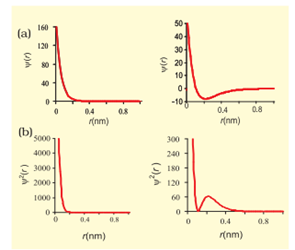Figure 1: The plots of (a) the orbital wave function ψ(r); (b) the variation of probability density ψ2 (r) as a function of distance r of the electron from the nucleus for 1s and 2s orbitals.

Boundary surface diagrams of the constant probability density for different atomic orbitals provides a good representation of the shapes of the atomic orbitals. In the figure, a boundary surface or contour surface is drawn in space for the orbital where the value of probability density |ψ| 2 is constant. The 1s and 2s orbital are spherical in shape but the s orbitals are in general spherical symmetric. This means that the probability of finding the electron at a given distance is equally uniform in all directions.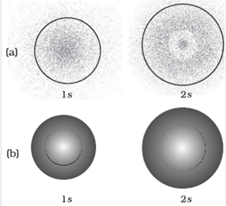Figure 2: (a) Probability density plots of 1s and 2s atomic orbitals. The density of the dots represents the probability density of finding the electron in that region. (b) Boundary surface representation for 1s and 2s orbitals.

### p Orbital

The p orbitals are dumbbell-shaped. The node in the p orbital occurs at the centre of the nucleus. The p orbital can occupy a maximum of six electrons due to the presence of three orbitals. The three p orbitals are oriented right angles to each other. The size of the p orbitals depends on the principal quantum number n i.e; 4p > 3p > 2p.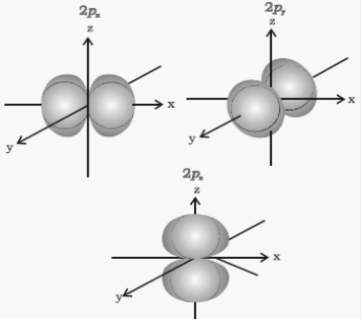Figure 3: Boundary surface diagrams of the three 2p orbitals.

Each p orbital has sections known as lobes which are present on either side of the plane which passes through the nucleus. The probability of finding an electron is zero at the plane where the two lobes intersect each other. The three orbital has identical size, shape and energy so-termed as degenerate orbitals. The orbitals only differ in the orientation of the lobes. The lobes are oriented along the x, y or z-axis thus are assigned with the designations 2px, 2py, and 2pz. The number of nodes is calculated by the formula n –2.

 Orbital px py pz m 0 Nodal plane xy yz zx

### d orbital

The d orbital is cloverleaf or two dumbbells in a plane. For d orbital the value of l=2 thus the minimum value of principal quantum number n is 3. The value for l cannot be greater than n-1. The values of ml corresponding to d orbital are (–2, –1, 0, +1 and +2) for l = 2 therefore, there are five d orbitals.

The five d-orbitals are assigned with the designation dxy, dyz, dxz, dx 2–y 2 and dz 2. The energy of all five orbitals are equal but the first four orbitals are similar in shape to each other while the dz2 differ from others. The radical node ( probability density function ) is zero. The dxy orbital has two nodal planes passing through the origin and bisects the xy plane consisting z-axis. There are two angular nodes for d orbital.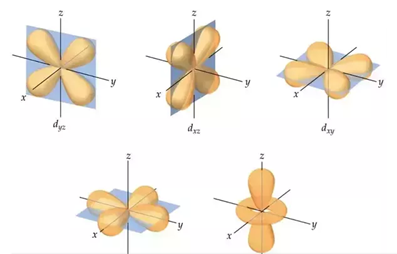Figure 4: Boundary surface diagrams of the five d orbitals.

 Orbital Nodal planes dxy xz, yz dyz xy, zx dzx xy, yz dx2-y2 x-y=0, x+y=0 dz2 No nodal plane

### f Orbitals

f orbital has diffused shape. For f orbital the value of l=3 thus the minimum value of principal quantum number n is 4. The values of ml corresponding to f orbital are (-3,–2, –1, 0, +1, +2, +3). For l = 3 therefore, there are seven f orbitals.

The seven orbitals are fx(x2-y2), fy(x2-y2), fxyz,fz3, fyz, fxz2,fz(x2-y2)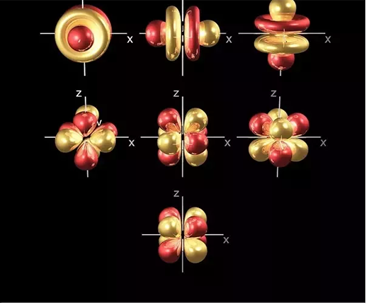Figure 5: Boundary surface diagrams of the seven f orbitals.

## Degenerate Orbitals

Degenerate orbitals are orbitals having the same energy. These orbital are different (may have a different orientation in space around the atomic nucleus) but possess the same energy. The degeneracy of p orbital remains unaffected in presence of external field but degeneracy of f and d orbital can be broken by applying an external field to the system (either electric or magnetic field).

Few orbitals will possess higher energy and other lower energy. The system will no longer have degeneracy. For example, d orbitals consist of five degenerated orbitals and all five orbitals have exactly the same energy.

Px,py,pz→ 3 fold degenerate

D orbitals → 5 fold degenerate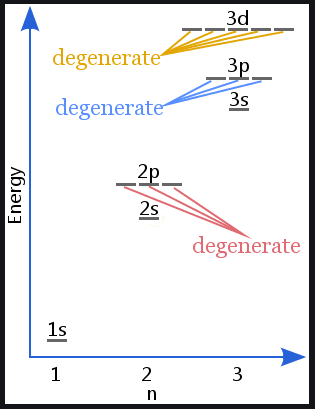Figure 6: Degenerate orbitals

## Atomic Structure Videos

### Atomic Structure – Orbitals, Orientation## Solved Problems

Question 1: Calculate the degeneracy of the level of the hydrogen atom having energy – RH / 25.

Solution:

The energy in H-atom, RH / n2

On comparing the energy, the value of n2 = 25

n = 5

The value of the azimuthal quantum number (l) is n-1

So the corresponding value of m is -l to +l

m=0, m=-1,0,1, m=-2,-1,0,1,2, m=-3,-2,-1,0,1,2,3, m=-4,-3,-2,-1,0,1,2,3,4

So the total number of orbitals are 1+3+4+7+9=25

The degeneracy of the energy levels of the hydrogen atom is 25

Question 2: Calculate the total spin resulting from a 3p4 configuration.

Solution:

The total number of unpaired electrons in 3p4 is 2

The total spin value is given by

Here, n is the number of unpaired electrons

The total spin resulting in 3p4 is 1.

Question 3: Determine the orbital angular momentum of an electron in 4f orbital.

Solution:

The orbital angular momentum =

$$\begin{array}{l}\sqrt{l (l+1)}\frac{h}{2\pi}\end{array}$$

The value of l corresponding to f orbital is 3

Thus the orbital angular momentum

$$\begin{array}{l}\sqrt{3 (3+1)}\frac{h}{2\pi}\end{array}$$
=
$$\begin{array}{l}\sqrt{12}\frac{h}{2\pi}\end{array}$$

The orbital angular momentum of an electron in 4f orbital is

$$\begin{array}{l}\sqrt{12}\frac{h}{2\pi}\end{array}$$
.

Question 4: If the value of magnetic quantum number m for an electron in an atom is equal to the number of m values for l =1. In which orbital the electron is present?

Solution:

The total values of m= (2l+1)

For l=1, the value of m is 21+1=3

m=3 for f subshell

So the electron is present in the f subshell.

Question 5: Which of the orbital has the shape of a baby-soother? And why?

Solution:

The dz2 orbital has the shape of a baby-soother because the orbital contains two lobes oriented in z-axis with the ring of high electron density concentrated in the xy plane. It contains no nodal plane rather has 2 nodal cones.

## Shapes of Orbitals and Nodes## Frequently Asked Questions on Orbitals

### What are the 4 prominent orbitals?

s, p, d, f are the four prominent atomic orbitals.

### What is the formula to calculate the number of radial nodes?

n-l-1 is the formula to calculate the number of radial nodes.

### What are the values of electron spin?

+½ and -½ are the two spin values of an electron.

### What is the shape of the p-orbital?

p-orbital has a dumb-bell shape.

### How many subshells does a d-orbital have?

The d-orbital has subshells dxy, dyz, dxz, dx2-dy2 and dz2.